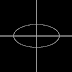In this tutorial you will learn how to draw an Ellipse on an image with PHP code. This is mostly used in Numerical Computing and Mathematics to draw graphs from algebraic functions and with standard equations.

```<?php
\$x = 600;
\$y = 315;
\$gd = imagecreatetruecolor(\$x, \$y);   //create background image
\$red = imagecolorallocate(\$gd, 255, 255, 255);   //create image
for (\$i = 0; \$i < 600; \$i++) {
imagesetpixel(\$gd, 300, \$i, \$red);  //draw vertical line
imagesetpixel(\$gd, \$i, 157, \$red);  //draw horizontal line
}
for(\$i = 1; \$i <= 360; \$i++)
{
\$x = 300 + 100 * cos(\$i);  //get x-axis with farmula
\$y = 157 + 50 * sin(\$i);   //get y-axis with farmula
imagesetpixel(\$gd, \$x, \$y, \$red);  //draw elips
}
PHP code to draw ellipse with Standard equation on an imageReviewed by Unknown on 22:56 Rating: 5